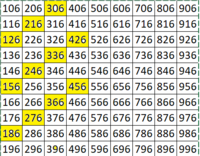# Three digit numbers which end in 6

#### apple2357

##### Full Member
Been working on a problem with my 11 year old. It was to find the probability that a three digit number ending in 6, will be a multiple of 6?

Intuitively what do you think this will be? Before reading on? 1/2, 1/4 ?

Anyway, the way we looked at it was to think about the first 10 or so 3 digit numbers which end in 6 - 106, 116, 126 etc and then look at the sum of the digits to see if they were a multiple of 6. We extended it into a grid, like so...We discovered there were 30 out of 90. So the probability was 1/3.

It felt a little unsatisfactory as we essentially had to search through a list, yes there is a pattern and it wasn't difficult to count but we basically counted them. Is there a quicker way this could be done? Feels like, given the answer comes to 1/3?

Looking beyond an 11 year old, i thought about using some algebra, so a 3 digit numbers abc could be written as 100a+10b+6 and this will be a multiple of 6 if a+b is a multiple of 3 . So i end up looking at solving a+b=3k, where a,b, k are natural numbers.
Suppose k=1: then a=1, b=2 or a=2, b=1
Suppose k=2: then a=1, b=5 or a=5, b=1 AND a=2, b=4 etc...

Again feels a little cumbersome?
Thoughts welcome.
My son then asked me how the probability varied for other last digits. I was confidently able to tell him that if it ended in a 2 it was a multiple of 2 (!).

Any thoughts?

#### lev888

##### Senior Member
The first 2 digits form a set of numbers. Which ones are divisible by 3? The answer is every third number.

•pepeketua, apple2357 and lex

#### lex

##### Junior Member

•apple2357

#### apple2357

##### Full Member
The first 2 digits form a set of numbers. Which ones are divisible by 3? The answer is every third number.
Thats exactly why i thought there was a quicker way! Thanks

#### Subhotosh Khan

##### Super Moderator
Staff member
Been working on a problem with my 11 year old. It was to find the probability that a three digit number ending in 6, will be a multiple of 6?

Intuitively what do you think this will be? Before reading on? 1/2, 1/4 ?

Anyway, the way we looked at it was to think about the first 10 or so 3 digit numbers which end in 6 - 106, 116, 126 etc and then look at the sum of the digits to see if they were a multiple of 6. We extended it into a grid, like so...

View attachment 26277

We discovered there were 30 out of 90. So the probability was 1/3.

It felt a little unsatisfactory as we essentially had to search through a list, yes there is a pattern and it wasn't difficult to count but we basically counted them. Is there a quicker way this could be done? Feels like, given the answer comes to 1/3?

Looking beyond an 11 year old, i thought about using some algebra, so a 3 digit numbers abc could be written as 100a+10b+6 and this will be a multiple of 6 if a+b is a multiple of 3 . So i end up looking at solving a+b=3k, where a,b, k are natural numbers.
Suppose k=1: then a=1, b=2 or a=2, b=1
Suppose k=2: then a=1, b=5 or a=5, b=1 AND a=2, b=4 etc...

Again feels a little cumbersome?
Thoughts welcome.
My son then asked me how the probability varied for other last digits. I was confidently able to tell him that if it ended in a 2 it was a multiple of 2 (!).
Any thoughts?
A number divisible by 6 must be:

• An even number (unit digit being 6 takes care of that)

• A number divisible by 3 → the digits added must be divisible by 3 (NOT by 6 as was stated in your OP)
The rule for divisibility by four:

A number like ......uvwxyz is divisible by 4​
if "yz" (a number made from last two digits - in the given order) is divisible 4​

Last edited:
•apple2357

#### apple2357

##### Full Member
So essentially
A number divisible by 6 must be:

• An even number (unit digit being 6 takes care of that)

• A number divisible by 3 → the digits added must be divisible by 3 (NOT by 6 as was stated in your OP)
The rule for divisibility by four:

A number like ......uvwxyz is divisible by 4​
if "yz" (a number made form last two digits - in the given order) is divisible 4​
Ah yes, well spotted!